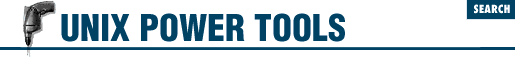home | O'Reilly's CD bookshelfs | FreeBSD | Linux | Cisco | Cisco Exam

#Chapter 47C Shell Programming...NOT## 47.3 Conditional Statements with if

.login and .cshrc files" 1070 is the use of conditionals ( if statements). This article explains the syntax of if statements. Article 47.4 of explains the syntax of the expressions you can test with an if .

The if command is used to begin a conditional statement. The simple format is:

```if (```

expr
```
) ```

cmd
```
```

There are three other possible formats, shown side-by-side:

```if (```

expr
```
) then   if (```

expr
```
) then   if (```

expr
```
) then
```

cmds
```
```

cmds1
```
```

cmds1
```

endif   else   else if (```

expr
```
) then
```

cmds2
```
```

cmds2
```

endif   else
```

cmds3
```

endif```

In the simplest form, execute ``` cmd ``` if ``` expr ``` is true; otherwise do nothing (redirection still occurs; this is a bug). In the other forms, execute one or more commands. If ``` expr ``` is true, continue with the commands after ``` then``` ; if ``` expr ``` is false, branch to the commands after ``` else``` (or after the ``` else if``` and continue checking). For example, the following if clause will take a default action if no command-line arguments are given:

```if (\$#argv == 0) then
echo "No filename given. Sending to Report."
set outfile = Report
else
set outfile = \$argv
endif```

For more examples, see article 47.4 .

- DG from O'Reilly & Associates' UNIX in a Nutshell (SVR4/Solaris)47.2 C Shell Programming Considered Harmful47.4 C Shell Variable Operators and Expressions